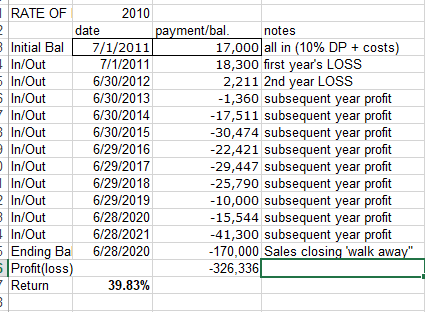# Help calculating IRR including sale proceeds

4 Replies

Hi, this is an excel question as much is it is an investment question, but I am wondering if folks here can provide any insight on how excel is taking cash flows as inputs.

I have series of cash flows in a single row. These are initial investment and yearly rental income.

When calculating IRR, my understanding is that we should include sale proceeds as part of the final year cash flow. This is a key assumption to my problem so if that is incorrect please let me know.

The excel formula of course uses the syntax IRR(values, guess)

For this example:
- Row 1 will hold yearly cash flow for years 1-5.
- Row 3 will hold sale proceeds
- I will sell the property in year 5

So it will look like this:

A1= 100 (cash outflow)
B1= 10
C1= 15
D1= 15
E1= 15
E3 = 25 (proceeds from sale of asset)

Here is the formula I am using to calculate this
=IRR((A1:E1,E3))

This will return a value but I am not sure if it is the correct way to calculate this because I am not sure how Excel is interpreting these flows with regard to timing.

I know that the extra set of () will allow you to use non-contiguous cells in the calculation and excel will see everything inside as a range of cells for the calculation.

If all the above is correct, my thought is that I should be adding the sale proceed to the final year cash flow from operating the property (E1+E3) and then using the result to calculate IRR.

If I try something like this is get an error: =IRR((A1:E1+E3))

With the first forumla above, my concern is that it is interpreting cell E3 as separate cash flow from a subsequent period, rather than occurring in the same period as the final operating cash flow.

This is minor distinction but I want to make sure I'm calculating this correctly.

I know I could add a new row that would hold the total cash flow (eg D4=D1+D3) and use D4 in the IRR calculation instead, but I feel like there should be a cleaner way.

Can someone set me straight on this? Thanks in advance.

@Brad E. Try using the XIRR function in excel instead of IRR

Ok, I will look in to that.  Thanks.

Originally posted by @Brad E. :

@Caleb Heimsoth

Ok, I will look in to that.  Thanks.

Let me know if you still have issues and I can walk you through what I did on my excel spread sheet a couple months agoi bought this building for 170K with 17K down in 2011, and had losses first two years. Then profits straight through. We are selling TODAY for 335K. Does the above copy and paste, using IRR function represent a pretty accurate view of my return? I am thinking that maybe I should combine first year's loss of 18~ with the down payment??

Thanks for any feedback. Different ways I've tweaked this all come pretty close. Up to 41% down to 37 and I just want to be sure I'm on the right track.